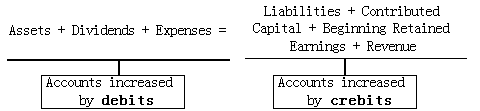Accounting equations
#has-images
Net income is equal to the income that a company has after subtracting costs and expenses from total revenue.

Revenue - Expenses = Net income (loss)

Net income is informally called the "bottom line" because it is typically found on the last line of a company's income statement.

Balance sheets and income statements are interrelated through the retained earnings component of owners' equity.

Ending retained earnings = Beginning retained earnings + Net income - Dividends

The following expanded accounting equation, which is derived from the above equations, provides a combined representation of the balance sheet and income statement:

Assets = Liabilities + Contributed capital + Beginning retained earnings + Revenue - Expenses - Dividends

• Dividends and expenses decrease owners' equity.
• Revenues increase owners' equity.

Because dividends and expenses are deductions from owners' equity, move them to the left side of the equation:If you want to change selection, open original toplevel document below and click on "Move attachment"